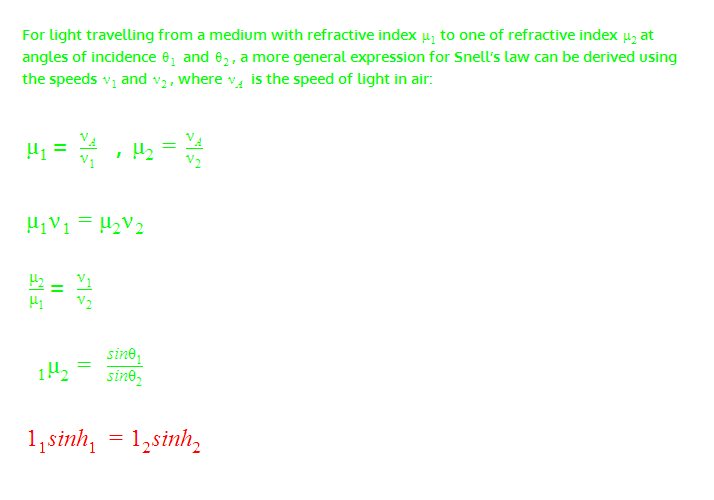# Sinh in Snell's Law?

hb20007
"sinh" in Snell's Law?

## Homework Statement

I'm trying to understand some equations in my AS level textbook about Snell's Law

## Homework Equations

The equations are in the image below

## The Attempt at a Solution

I tried reading up about sinh but I don't fully understand what it is. Also I'm not sure what the term beside sinh is. It can't be a "1" and to seems like a small letter L which comes out of nowhere?## Answers and Replies

Mentor
That looks like a typesetting problem. The greek letter θ corresponds to the roman letter q (try typing q in a font like Symbol). I'm not sure where the big 1 comes from, but it should be a μ. Then you recover Snell's law:
$$\mu_1 \sin \theta_1 = \mu_2 \sin \theta_2$$

Mentor
I have seen a version of Snell's law where there is not just refraction taking place but also energy absorption and reflection at interfaces. They used complex indexes of refraction and complex angles! Hyperbolic sines replaced the sin() functions. Sorry I don't remember the reference, it was a good many years ago!

Homework Helper
Snell's Law is equivalent to the continuity of the tangential component of the wave vector at an interface: The component of the wave vector k parallel to the surface is the same in both media. In case of an absorbing medium, k is complex. Representing the parallel component with ksinθ, Snells Law states that k1sinθ1=k2sinθ2.
If the light came from a non-absorbing medium, k1 and θ1 are real. If k2 is complex, θ2 is a complex angle, and you have the complex sine function, ##\sin(z)=\frac{\exp(iz)-\exp(-iz)}{2i}=-i \sinh(iz)## But you can avoid by computing with complex angles if you use Snell's Law in the form kparallel= ko sinθo.

ehild

Last edited:
Mentor
From the material presented in the OP, it is clear that this is introductory level. Hyperbolic sines have nothing to do with the poster's question, and the equation doesn't make mathematical sense (in terms of notation) if one interprets sinh as a hyperbolic sine.

Well, never trust texts that are written with M\$ Word or similar pseudo-typesetting programs. There are exceptions, but usually one has to be very careful with such texts.